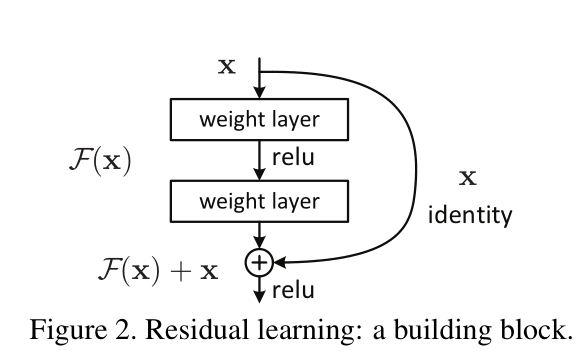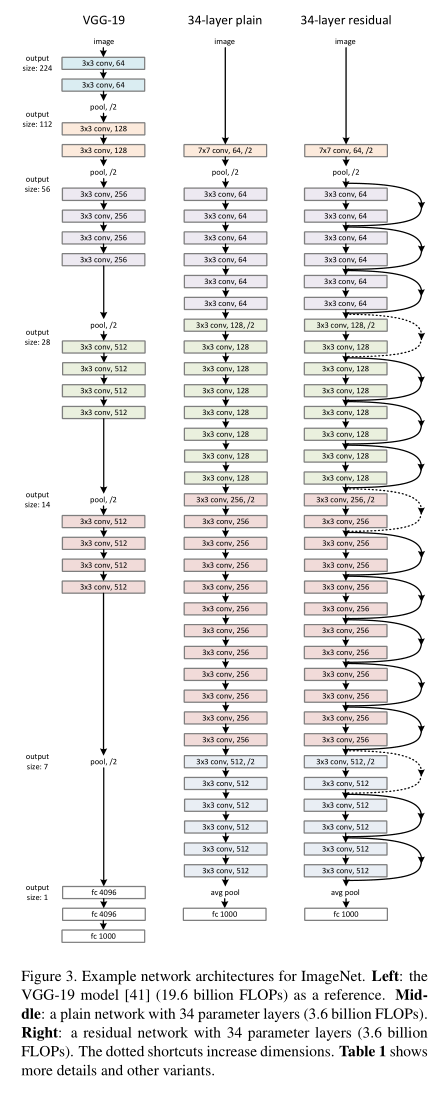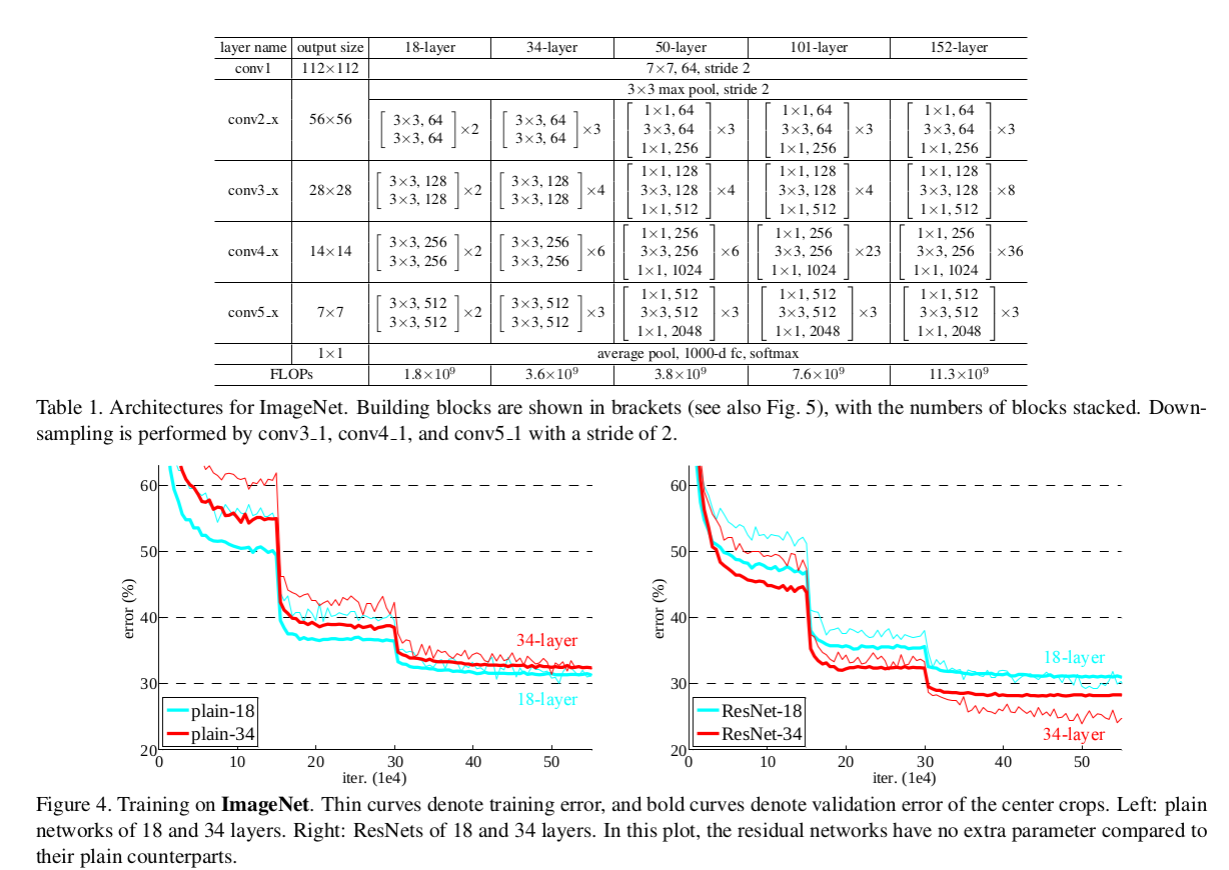## Friday, October 18, 2019

### ResNets inner workings and notes

A residual network (or ResNet) is a standard deep neural net architecture, with state-of-the-art performance across numerous applications. The main premise of ResNets is that they allow the training of each layer to focus on fitting just the residual of the previous layer’s output and the target output. Thus, we should expect that the trained network is no worse than what we can obtain if we remove the residual layers and train a shallower network instead.

Up until 2015 we had 3 mainstream ways of training deep networks:
• Greedy per layer optimization and freezing
• Various flavors of Dropout
• and Batch Normalization (came out early 2015, wasn't mainstream until 2016, now it is patented by google so who knows if people can use it)
The main contribution of the "Deep Residual Learning for Image Recognition, 2015" paper is a novel  and smart building block for training very deep neural networks.

### The "Residual Learning" or "Identity Learning" block.A special note here:
We need at least 2 weight layers here (nonlinearities are not essential but welcome) to get the benefits of the universal function approximator that has been proven since the 90's. You can add more but you then hit the limits of information propagation and you will need residual/skip connections inside the residual block.

A stack of $$n$$ residual blocks is described as follows :

$$x_0$$ : input
$$x_1 = x_0 + F_1(x_0)$$
$$x_2 = x_1 + F_2(x_1)$$
$$x_3 = x_2 + F_3(x_2)$$
$$...$$
$$x_n = x_{n-1} + F_{n-1}(x_{n-1})$$

This can be re-written as  :

$$x_n = x_{n-1} + F_{n-1}( x_{n-2} + F_{n-2}(x_{n-2})$$

Which can be expanded as :

$$x_n = x_{n-1} + F_{n-1}( x_{n-2} + F_{n-2}( x_{n-3} +F_{n-3}( x_{n-4} + F_{n-4}(... + F_1(x_0))))))$$

Now if we assume that $$F_i$$ is a linear function (it is not for $$x \lt 0$$ ) but we can ignore it) :

$$x_n = x_{n-1} + F_{n-1}( x_{n-2} ) + F_{n-1}F_{n-2}(x_{n-3}) + F_{n-1}F_{n-2}F_{n-3}(x_{n-4}) + ... F_{n-1}F_{n-2}F_{n-3}...F_1(x_0)$$

As we can see from the equations and the equivalent graph, there is a clear information flow from the raw data to the output. This means that the major pain point of vanishing gradient is avoided.This design when stacked as above allows the gradient to flow through the whole network bypassing any tricky points and in essence training the deeper network properly. Information bottlenecks can be introduced by design but the gradient can flow from the loss layer up to the base layer.

Residual Networks (ResNets) have been used to train up to a 1000 layers. It was proven empirically that going deeper generalizes better than shallow wide networks. Again empirically several variations of the Residual Block are tried:In practice the Residual Function is not 2 (convs or fully connected) weight layers but they are accompanied by a Batch Normalization layer. This helps stabilize the gradient and the co-variate shift (there are various opposing hypothesis on why batch norm works).

In the paper "Identity Mappings in Deep Residual Networks, 2016" a different layout was proposed that improved the performance. We can see an inversion of the order of the layers. The architecture, the analysis and the system design remains the same though.
This new arrangements produces better results on very deep networks for the CIFAR dataset, shown below. Also the convergence is much faster. Since they are practically the same from an engineering perspective we don't have a reason to reject the one with the better performance.